# 题目

1. 行人身体表面将淋多少雨？

2. 行人应该用多大速度回家才能淋最少的雨？

3. 假如奔跑方向有风，风速为Vw，此时应以多大速度奔跑？

4. 请设计合理的方法来测定雨的下落速度、雨水打湿面积百分比、人的正投影面积和侧投影面积，并给出各类天气下不同距离的奔跑速度建议。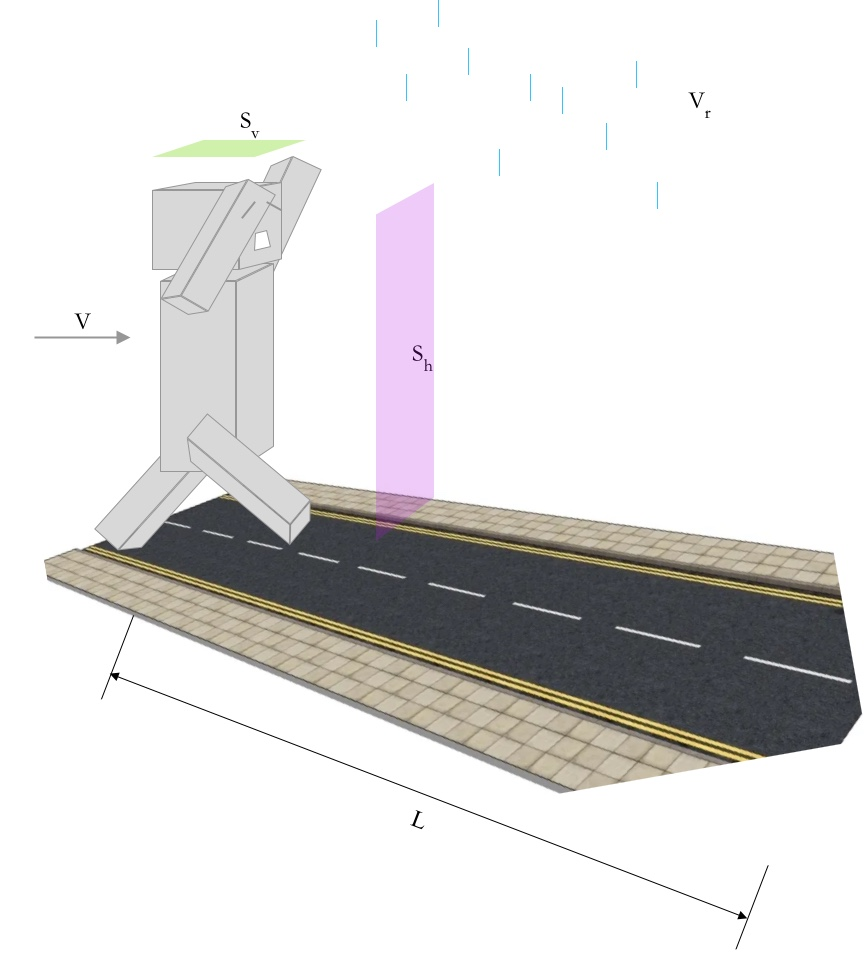# 3模型建立与求解

## 3.1针对问题1和2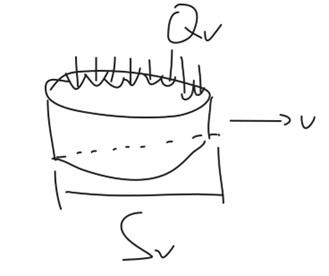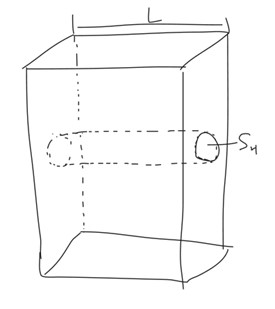$$Q(V) = \frac{L}{V}{V_w}{V_r}{S_v} + L{S_h}{V_W}$$

## 3.2针对问题3

$$Q(V) = \frac{{{V_W}L}}{V}[{V_r}{S_v} + {V_s}{S_s} + |v - {v_n}|{S_h}]$$

$$\frac{{dQ}}{{dV}} = {V_w}[ - \frac{L}{{{v^2}}}({v_r}{S_v} + {v_a}{S_s} - {v_n}{S_h})],v - {v_n} > 0$$
$$\frac{{dQ}}{{dV}} = {V_w}[ - \frac{L}{{{v^2}}}({v_r}{S_v} + {v_a}{S_s} - {v_n}{S_h})],v - {v_n} < 0$$

$$=> {v_{cr}} = \pm \frac{{{v_r}{S_v} + {v_a}{S_s}}}{{{S_h}}}$$

$$=> {v_{cr}} = \frac{{{v_r}{S_v} + {v_a}{S_s}}}{{{S_h}}}$$

1. 当$v_n$大于$v_{cr}$时，风速由顺风风速不断增大，则Q变成了增函数，随着v的增大而增大；
2. 当$v_n$小于$v_{cr}$时，此时顺风风速不断减小，渐渐转变为逆风，此时Q为减函数，随着v的增大而减小；
3. 当$v_n$等于$v_{cr}$时，淋雨量导数恒为0，即当奔跑速度满足大于$v_n$的条件时，淋雨量与奔跑速度无关。

$$=> {v_{cr}} = - \frac{{{v_r}{S_v} + {v_a}{S_s}}}{{{S_h}}}$$

1. 当$v_n$大于$v_{cr}$时，风速由顺风不断增大，Q变为了减函数，随着v的增大而减小；
2. 当$v_n$小于$v_{cr}$时，风速由顺风不断减小，直到逆风，Q变为了增函数，随着v的而增大；
3. 当$v_n$等于$v_{cr}$时，淋雨量导数恒为0，即当奔跑速度满足大于0小于$v_n$的条件时，淋雨量与奔跑速度无关。

1. 存在一个临界风速$v_{cr}$使得人奔跑时候的淋雨量与人的运动速度无关；
2. 当人奔跑的速度v较小时，如实际风速小于临界风速，淋雨量随着奔跑的速度的增大而增大；反之则减小；
3. 当人奔跑的速度v较大时，实际风速小于临界封堵，淋雨量随着奔跑的速度增大而减小，反之则增大。 因此人在决定奔跑前应该首先测量一下风速，如果风速较大且顺风的话，应该尽可能地跑快；如果风速较小且顺风的话应该奔跑速度应该保持和风速差不多。如果风速较大为逆风的话，人应该尽可能地跑快。

## 3.3针对问题4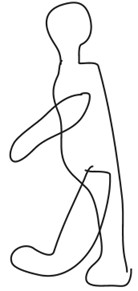# 4模型的进一步改进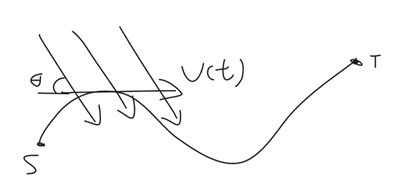$${v_a} = {v_w}\cos (\theta (t) - \varphi (t))$$

$${v_n} = {v_w}\sin (\theta (t) - \varphi (t))$$

$$Q(v) = {V_w}\int_0^{{t_0}} {{v_r}{S_v} + {v_a}{S_s} + |v - {v_n}|{S_h}dt}$$

https://www.eatrice.cn/post/KeepWalkingInTheRain/

2022年6月7日